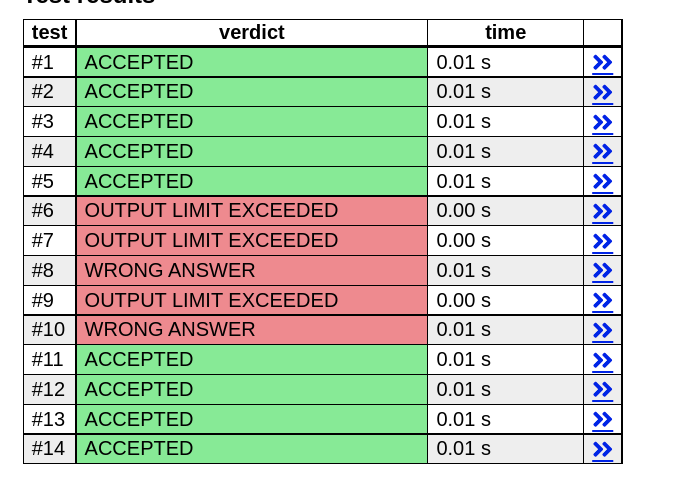Hi,

I have just started using the USACO Guide, and I was working on the CSES problem set. I tried to submit my code on cses, and got the following solution:

``````    #include <bits/stdc++.h>
using namespace std;

const long long max_n = 1000000;
long long n;

void solve(int n) {
cout << n;

while(n != 1){
if(n % 2 == 0) n /= 2;
else n = 3 * n + 1;

cout << " " << n;
}

cout << endl;

return;
}

int main() {
ios::sync_with_stdio(false);
cin.tie(NULL);

cin >> n;
solve(n);
}
``````

The CSES: